It is currently Sun May 26, 2019 8:41 am

 All times are UTC - 8 hours [ DST ]

 Print view Previous topic | Next topic
Author MessagePost subject: Hyperactive Kos#1 Posted: Sat Dec 15, 2018 3:02 am
 Tengen

Posts: 4722
Liked others: 0
Was liked: 644
How to calculate counts and move values of this hyperactive ko locally with komaster case analysis?

Click Here To Show Diagram Code
`[go]\$\$B \$\$ ---------------------------\$\$ . X X . . X O . O . . O O .\$\$ . X . O O . X O . X X . O .\$\$ . X . O O . X O . X X . O .\$\$ . X X X X X X O O O O O O .[/go]`

The following easier positions I think I can calculate:

Click Here To Show Diagram Code
`[go]\$\$B \$\$ ---------------------------\$\$ . X X . . X O . O . . O O .\$\$ . X . O O X X O . X X . O .\$\$ . X . O O . X O . X X . O .\$\$ . X X X X X X O O O O O O .[/go]`

Click Here To Show Diagram Code
`[go]\$\$B \$\$ ---------------------------\$\$ . X X . . X O . O . . O O .\$\$ . X . O O . X O X X X . O .\$\$ . X . O O . X O . X X . O .\$\$ . X X X X X X O O O O O O .[/go]`

What is an example of an active (not hyperactive) ko?

TopPost subject: Re: Hyperactive Kos#2 Posted: Tue Dec 18, 2018 9:05 am
 Honinbo

Posts: 8404
Liked others: 2448
Was liked: 2909
RobertJasiek wrote:
What is an example of an active (not hyperactive) ko?

Hyperactive kos are those whose mast values (counts) depend upon who is komaster. I coined the term, active ko, for those kos whose mast values remain the same under different komaster conditions, but whose temperatures (miai values) depend upon who is komaster. Active kos are rare. Kos whose mast values and temperatures remain the same, regardless of who is komaster, are call placid.

Next I will do an analysis of this complicated ko position that is doable at the table._________________
There is one human race.
----------------------------------------------------

The Adkins Principle:

At some point, doesn't thinking have to go on?

— Winona Adkins

TopPost subject: Re: Hyperactive Kos#3 Posted: Tue Dec 18, 2018 10:15 am
 Honinbo

Posts: 8404
Liked others: 2448
Was liked: 2909
RobertJasiek wrote:
How to calculate counts and move values of this hyperactive ko locally with komaster case analysis?

Click Here To Show Diagram Code
`[go]\$\$B \$\$ ---------------------------\$\$ . X X . . X O . O . . O O .\$\$ . X . O O . X O . X X . O .\$\$ . X . O O . X O . X X . O .\$\$ . X X X X X X O O O O O O .\$\$ . . . . . . . . . . . . . .[/go]`

This is a complicated position. I assume that if surrounded stones are cut off, they are dead. And, per convention, that the outer stones are alive.

Because of the symmetrical nature of this position, let's just do the calculations for White komaster. From those we can discern the values when Black is komaster.Click Here To Show Diagram Code
`[go]\$\$W White first\$\$ ---------------------------\$\$ . X X . 3 X O . O . . O O .\$\$ . X . O O 1 X O . X X . O .\$\$ . X . O O . X O . X X . O .\$\$ . X X X X X X O O O O O O .\$\$ . . . . . . . . . . . . . .[/go]`

When White is komaster he can make a large ko with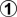and win it. (Probably not right away, OC, but we can skip the ko fight.) The result is a local score of -15 (15 pts. for White).

To find the mast value and temperature we have to find Black's threat. Next is one possibility.

Click Here To Show Diagram Code
`[go]\$\$B Black first\$\$ ---------------------------\$\$ . X X . . X O 1 O 5 . O O .\$\$ . X . O O . X O 3 X X . O .\$\$ . X . O O . X O . X X . O .\$\$ . X X X X X X O O O O O O .\$\$ . . . . . . . . . . . . . .[/go]`

In three moves Black can take the ko, make the larger ko, and win it. OC, White will prevent this from happening, but this is one threat. The resulting local score is 16 pts. (for Black).

Assuming that this is Black's threat, there is a swing of 31 pts. in 5 moves, for an average gain of 6.2 pts. per move.

However,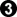actually makes a larger ko by giving up an extra stone when White wins the ko, for a swing of 32 pts. in 4 moves, or an average gain of 8 pts. per move. (Edited for correctness after Robert pointed out that I miscounted the net number of moves.)

That makessente, so White will take and win the resulting larger ko.

Click Here To Show Diagram Code
`[go]\$\$B Black first\$\$ ---------------------------\$\$ . X X . 8 X W 1 O . . O O .\$\$ . X . O O 6 X O 3 X X . O .\$\$ . X . O O . X O . X X . O .\$\$ . X X X X X X O O O O O O .\$\$ . . . . . . . . . . . . . .[/go]`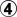@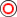If Black cuts withWhite can take the ko back, make a larger ko and win it for a score of -16 pts. in one net play, instead of getting a score -15 pts. in two net plays. It isn't just thatloses one point, it loses one play, as well.is a mistake.

But Black has another possible threat. Let's look at it.

Click Here To Show Diagram Code
`[go]\$\$B Black first\$\$ ---------------------------\$\$ . X X . . X W 1 O . . O O .\$\$ . X . O O . X O a X X . O .\$\$ . X . O O . X O . X X . O .\$\$ . X X X X X X O O O O O O .\$\$ . . . . . . . . . . . . . .[/go]`@Since White is komaster, simply filling the ko is correct. If Black later cuts at "a" the result will be 16 pts., as we know. If White connects at "a" the result will be 2 pts. So in this position the count is 9 pts. and each play gains 7 pts. Since 7 > 6.2 we expect thatatis sente. (Edited for correctness, as above.)

Let's check that. White to play can play to a position worth -15 pts. in two moves, while Black to play threatens to play to a position worth 2 pts. in one net play. The swing is 17 pts. in three net plays, for an average gain per play of 5⅔ pts., which is less than 7 pts. Soatis sente.

We now take this to be Black's threat (showing the sente).

Click Here To Show Diagram Code
`[go]\$\$B Black first\$\$ ---------------------------\$\$ . X X . . X W 1 O . . O O .\$\$ . X . O O . X O 4 X X . O .\$\$ . X . O O . X O . X X . O .\$\$ . X X X X X X O O O O O O .\$\$ . . . . . . . . . . . . . .[/go]`@But Black has another threat, which also looks obvious.

Click Here To Show Diagram Code
`[go]\$\$B Black first\$\$ ---------------------------\$\$ . X X . . X O . O . . O O .\$\$ . X . O O 1 X O . X X . O .\$\$ . X . O O . X O . X X . O .\$\$ . X X X X X X O O O O O O .\$\$ . . . . . . . . . . . . . .[/go]`

Since White is komaster, Black can capture the White stones with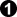, leaving a small ko. Let's analyze it, with White komaster.

Click Here To Show Diagram Code
`[go]\$\$W White first\$\$ ---------------------------\$\$ . X X . . X O 1 O . . O O .\$\$ . X . O O B X O . X X . O .\$\$ . X . O O . X O . X X . O .\$\$ . X X X X X X O O O O O O .\$\$ . . . . . . . . . . . . . .[/go]`

White to play can simply fill the ko, for a local score of -1 pt.

Click Here To Show Diagram Code
`[go]\$\$B Black first\$\$ ---------------------------\$\$ . X X . . X W 1 O . . O O .\$\$ . X . O O B X O 4 X X . O .\$\$ . X . O O . X O . X X . O .\$\$ . X X X X X X O O O O O O .\$\$ . . . . . . . . . . . . . .[/go]`@OC,atis Black's threat, and is sente. The result is a local score of 1 pt., after 1 net play.

There is a swing of 2 pts. in 2 net plays, for an average gain per play of 1 pt. So the local count after Black connects at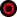is 0, with a local temperature of 1 pt. By comparison Black's threat to take and connect the ko with sente reaches a local score of 2 pts., and is the better threat. This result may seem counterintuitive.

To summarize:

When White is komaster,

Click Here To Show Diagram Code
`[go]\$\$W White first\$\$ ---------------------------\$\$ . X X . 3 X O . O . . O O .\$\$ . X . O O 1 X O . X X . O .\$\$ . X . O O . X O . X X . O .\$\$ . X X X X X X O O O O O O .\$\$ . . . . . . . . . . . . . .[/go]`

White to play cuts atand wins the resulting ko in two net plays, for a local score of -15.

Click Here To Show Diagram Code
`[go]\$\$B Black first\$\$ ---------------------------\$\$ . X X . . X W 1 O . . O O .\$\$ . X . O O . X O 4 X X . O .\$\$ . X . O O . X O . X X . O .\$\$ . X X X X X X O O O O O O .\$\$ . . . . . . . . . . . . . .[/go]`@Black's threat is to take the ko and fill it with sente, for a local score of 2 pts. in one net play.

The swing is 17 pts. in three net plays, for an average gain of 5⅔ pts. per play. The local count is -3⅔ pts.

Edited for correctness and clarity.Later edited again for correctness.

Edit:

When Black is komaster:

Click Here To Show Diagram Code
`[go]\$\$B \$\$ ---------------------------\$\$ . X X . . X O . O . . O O .\$\$ . X . O O . X O . X X . O .\$\$ . X . O O . X O . X X . O .\$\$ . X X X X X X O O O O O O .\$\$ . . . . . . . . . . . . . .[/go]`

This position is a White sente with a count of -1. OC, each net play in the ko gains on average 5⅔ pts.Who is komaster makes a difference of 2⅔ pts. in the count.

_________________
There is one human race.
----------------------------------------------------

The Adkins Principle:

At some point, doesn't thinking have to go on?

— Winona Adkins

Last edited by Bill Spight on Wed Dec 19, 2018 5:52 pm, edited 1 time in total.
TopPost subject: Re: Hyperactive Kos#4 Posted: Wed Dec 19, 2018 12:50 am
 Tengen

Posts: 4722
Liked others: 0
Was liked: 644
Bill Spight wrote:
Assuming that this is Black's threat, there is a swing of 31 pts. in 5 moves, for an average gain of 6.2 pts. per move.

However, :b3: actually makes a larger ko by giving up an extra stone when White wins the ko, for a swing of 32 pts. in 5 moves, or an average gain of 6.4 pts. per move.

That makes :b3: sente, so White will take and win the resulting larger ko.

This kind of comparison of, and decision-making between, two variations I was missing, many thanks!

We can calculate the average gain of either variation because there is only one sequence started by White. In other initial positions, can it happen that there would be two or more sequences started by White to be considered? If yes, how to calculate each average gain, compare and decide between variations?

Why do you speak of "threat"? Is it not easier to speak of choice, strategy or tactical variation?

Why do you speak of "sente"? What is "sente" in a tree of a local ko endgame? Just an informal word to express that a player's local play is correctly answered by the opponent's next move being a local play?

Quote:
However, :b3: actually makes a larger ko by giving up an extra stone when White wins the ko, for a swing of 32 pts. in 5 moves

Let me try to understand how to calculate the tally and after what sequences' followers to calculate the swing:

Click Here To Show Diagram Code
`[go]\$\$B initial sequence\$\$ ---------------------------\$\$ . X X . . X 4 1 O . . O O .\$\$ . X . O O . X O 3 X X . O .\$\$ . X . O O . X O . X X . O .\$\$ . X X X X X X O O O O O O .\$\$ . . . . . . . . . . . . . .[/go]`

Initial prisoner difference = 0.

Initial excess plays = 1.

Click Here To Show Diagram Code
`[go]\$\$B Black continues\$\$ ---------------------------\$\$ . X X . . X O 5 O 7 . O O .\$\$ . X . O O . X O X X X . O .\$\$ . X . O O . X O . X X . O .\$\$ . X X X X X X O O O O O O .\$\$ . . . . . . . . . . . . . .[/go]`

Black continuation's excess plays = 2.

Black follower's count including the initial prisoner difference = 16 + 0 = 16.

Click Here To Show Diagram Code
`[go]\$\$B White continues\$\$ ---------------------------\$\$ . X X . 8 X O . O . . O O .\$\$ . X . O O 6 X O X X X . O .\$\$ . X . O O . X O . X X . O .\$\$ . X X X X X X O O O O O O .\$\$ . . . . . . . . . . . . . .[/go]`

White continuation's excess plays = 2.

White follower's count including the initial prisoner difference = -16 + 0 = -16.

Total excess plays = initial excess plays + Black continuation's excess plays + White continuation's excess plays = 1 + 2 + 2 = 5.

Swing = 16 + (-16) = 32.

Is this calculation right?

If yes, why does White being the komaster allow Black 5 in Black's continuation?

If no, how to calculate the tally, determine the sequences to what followers so that Black 3 occurs, and correctly calculate the prisoner difference?

Quote:
Click Here To Show Diagram Code
`[go]\$\$B Black first\$\$ ---------------------------\$\$ . X X . 8 X W 1 O . . O O .\$\$ . X . O O 6 X O 3 X X . O .\$\$ . X . O O . X O . X X . O .\$\$ . X X X X X X O O O O O O .\$\$ . . . . . . . . . . . . . .[/go]`

:w4: @ :wc:

If Black cuts with :b3: White can take the ko back, make a larger ko and win it for a score of -16 pts. in one net play, instead of getting a score -15 pts. in two net plays. It isn't just that :b3: loses one point, it loses one play, as well. :b3: is a mistake.

I understand this but...

a) Black starts this sequence. Why may we compare it to the sequence started by White to assess that the former has the mistake Black 3?

b) If we use this reasoning in your text to identify the mistake Black 3, why do we need the following cited analysis?

Quote:
Click Here To Show Diagram Code
`[go]\$\$B Black first\$\$ ---------------------------\$\$ . X X . . X O 1 O 5 . O O .\$\$ . X . O O . X O 3 X X . O .\$\$ . X . O O . X O . X X . O .\$\$ . X X X X X X O O O O O O .\$\$ . . . . . . . . . . . . . .[/go]`

In three moves Black can take the ko, make the larger ko, and win it. OC, White will prevent this from happening, but this is one threat. The resulting local score is 16 pts. (for Black).

Assuming that this is Black's threat, there is a swing of 31 pts. in 5 moves, for an average gain of 6.2 pts. per move.

However, :b3: actually makes a larger ko by giving up an extra stone when White wins the ko, for a swing of 32 pts. in 5 moves, or an average gain of 6.4 pts. per move.

That makes :b3: sente, so White will take and win the resulting larger ko.

Other topic:

Quote:
Click Here To Show Diagram Code
`[go]\$\$B Black first\$\$ ---------------------------\$\$ . X X . . X W 1 O . . O O .\$\$ . X . O O . X O 4 X X . O .\$\$ . X . O O . X O . X X . O .\$\$ . X X X X X X O O O O O O .\$\$ . . . . . . . . . . . . . .[/go]`

:b3: @ :wc:

Although White is the komaster, White 2 may not recapture due to the ko rule. White need not play 2 at 4 because he is the komaster and can force Black to first play at 3. Therefore, White's correct local move 2 is a pass.

However, if White plays 2 at 4, Black's correct play in view of White being the komaster is to connect the ko. The resulting count is the same. IOW, the diagram shows a dominating White 2.

***

Now, we might also analyse the case "neither player is komaster".

***

While I am approaching an understanding of analysis of this example, the general method remains a mystery for me.

***

On Sensei's and here, you define active ko. I understand that it is rare. Uh. So rare that you do not know any example, can you show some or is it top secret?

TopPost subject: Re: Hyperactive Kos#5 Posted: Wed Dec 19, 2018 8:35 am
 Honinbo

Posts: 8404
Liked others: 2448
Was liked: 2909
RobertJasiek wrote:
Bill Spight wrote:
Assuming that this is Black's threat, there is a swing of 31 pts. in 5 moves, for an average gain of 6.2 pts. per move.

However,actually makes a larger ko by giving up an extra stone when White wins the ko, for a swing of 32 pts. in 5 moves, or an average gain of 6.4 pts. per move.

That makessente, so White will take and win the resulting larger ko.

Why do you speak of "sente"? What is "sente" in a tree of a local ko endgame? Just an informal word to express that a player's local play is correctly answered by the opponent's next move being a local play?

You may consider "sente" to be informal here, but in the context of kos the term can acquire new technical meanings, if one bothers to define them. In this case, raising the temperature is part of the meaning. Because White is komaster, we already know that White's answer is correct play.

RobertJasiek wrote:
We can calculate the average gain of either variation because there is only one sequence started by White. In other initial positions, can it happen that there would be two or more sequences started by White to be considered? If yes, how to calculate each average gain, compare and decide between variations?

You are asking a lot!As is well established, non-ko positions may be solved (in the sense of finding their mean values and temperatures) by using the method of multiples to find mean values (counts), from which miai values (temperatures) may be derived. They may also be solved using thermography. You can even apply the method of multiples to placid ko positions. But hyperactive kos require thermographic ideas to solve. That is, correct local play depends crucially upon the environment in a way that the method of multiples does not address. For one thing, with hyperactive kos we do not speak of mean values, but of mast values. The mast, OC, is a thermographic concept.

The approach which I have taken here is top down, to attempt to find the temperature (average gain per play) above which at least one player will prefer to play elsewhere (assuming an ideal environment). If the position is too complicated to do that and proceed top down, the task becomes more difficult and laborious.

RobertJasiek wrote:
Why do you speak of "threat"? Is it not easier to speak of choice, strategy or tactical variation?

I speak of Black's threat because we do not expect Black, as koloser, to be able to carry it out. But we need to know it because we need to know when it is too small for White to prevent it. Assuming that we have found White's correct play and Black's correct threat, when the average gain of plays elsewhere (in the environment) is equal to the average gain of the ko plays, White will be indifferent between preventing Black's threat or not.

The concept of threat helps to explain the discussion below.RobertJasiek wrote:
Quote:
However,actually makes a larger ko by giving up an extra stone when White wins the ko, for a swing of 32 pts. in 5 moves

Let me try to understand how to calculate the tally and after what sequences' followers to calculate the swing:

Click Here To Show Diagram Code
`[go]\$\$B initial sequence\$\$ ---------------------------\$\$ . X X . . X 4 1 O . . O O .\$\$ . X . O O . X O 3 X X . O .\$\$ . X . O O . X O . X X . O .\$\$ . X X X X X X O O O O O O .\$\$ . . . . . . . . . . . . . .[/go]`

If you want to calculate the swing you have to let Black finish her threat.

Quote:
Initial prisoner difference = 0.

Initial excess plays = 1.

Click Here To Show Diagram Code
`[go]\$\$B Black continues\$\$ ---------------------------\$\$ . X X . . X O 5 O 7 . O O .\$\$ . X . O O . X O X X X . O .\$\$ . X . O O . X O . X X . O .\$\$ . X X X X X X O O O O O O .\$\$ . . . . . . . . . . . . . .[/go]`

There is potential for confusion here. As koloser, Black should not be able to take the ko back.

But you did finally let Black complete her threat.Quote:
Black continuation's excess plays = 2.

Black follower's count including the initial prisoner difference = 16 + 0 = 16.

Click Here To Show Diagram Code
`[go]\$\$B White continues\$\$ ---------------------------\$\$ . X X . 8 X O . O . . O O .\$\$ . X . O O 6 X O X X X . O .\$\$ . X . O O . X O . X X . O .\$\$ . X X X X X X O O O O O O .\$\$ . . . . . . . . . . . . . .[/go]`

Black did not take back with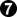. Good.Quote:
White continuation's excess plays = 2.

White follower's count including the initial prisoner difference = -16 + 0 = -16.

Total excess plays = initial excess plays + Black continuation's excess plays + White continuation's excess plays = 1 + 2 + 2 = 5.

Swing = 16 + (-16) = 32.

Is this calculation right?

Yes.

Quote:
If yes, why does White being the komaster allow Black 5 in Black's continuation?

To discover Black's threat.

But, technically, having taken the ko with, as komaster White should not allow Black to take the ko back.

RobertJasiek wrote:
Quote:
Click Here To Show Diagram Code
`[go]\$\$B Black first\$\$ ---------------------------\$\$ . X X . 8 X W 1 O . . O O .\$\$ . X . O O 6 X O 3 X X . O .\$\$ . X . O O . X O . X X . O .\$\$ . X X X X X X O O O O O O .\$\$ . . . . . . . . . . . . . .[/go]`@If Black cuts withWhite can take the ko back, make a larger ko and win it for a score of -16 pts. in one net play, instead of getting a score -15 pts. in two net plays. It isn't just thatloses one point, it loses one play, as well.is a mistake.

I understand this but...

a) Black starts this sequence. Why may we compare it to the sequence started by White to assess that the former has the mistake Black 3?

Because the comparison shows thatis worse than a pass.

RobertJasiek wrote:
b) If we use this reasoning in your text to identify the mistake Black 3, why do we need the following cited analysis?

Quote:
Click Here To Show Diagram Code
`[go]\$\$B Black first\$\$ ---------------------------\$\$ . X X . . X O 1 O 5 . O O .\$\$ . X . O O . X O 3 X X . O .\$\$ . X . O O . X O . X X . O .\$\$ . X X X X X X O O O O O O .\$\$ . . . . . . . . . . . . . .[/go]`

In three moves Black can take the ko, make the larger ko, and win it. OC, White will prevent this from happening, but this is one threat. The resulting local score is 16 pts. (for Black).

Assuming that this is Black's threat, there is a swing of 31 pts. in 5 moves, for an average gain of 6.2 pts. per move.

However,actually makes a larger ko by giving up an extra stone when White wins the ko, for a swing of 32 pts. in 5 moves, or an average gain of 6.4 pts. per move.

That makessente, so White will take and win the resulting larger ko.

We do not actually need it. However, since we are working top down and trying to find Black's best threat, we initially allowand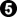. Having done so, this line of reasoning enables us to discover thatis a mistake.

RobertJasiek wrote:
Other topic:

Quote:
Click Here To Show Diagram Code
`[go]\$\$B Black first\$\$ ---------------------------\$\$ . X X . . X W 1 O . . O O .\$\$ . X . O O . X O 4 X X . O .\$\$ . X . O O . X O . X X . O .\$\$ . X X X X X X O O O O O O .\$\$ . . . . . . . . . . . . . .[/go]`@Although White is the komaster, White 2 may not recapture due to the ko rule. White need not play 2 at 4 because he is the komaster and can force Black to first play at 3. Therefore, White's correct local move 2 is a pass.

I believe this comes from my analysis.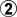plays elsewhere because we want to discover Black's threat. Otherwise he would play his sufficiently large threat and take the ko back.

RobertJasiek wrote:
However, if White plays 2 at 4, Black's correct play in view of White being the komaster is to connect the ko. The resulting count is the same. IOW, the diagram shows a dominating White 2.

Ifis at 4, the local temperature has dropped and Black should pass.

RobertJasiek wrote:
***

Now, we might also analyse the case "neither player is komaster".

***

If neither player is komaster, whoever wins the ko fight must ignore a ko threat to do so. That means that the properties of ko threats become significant. There is no single way to handle the no komaster case for non-placid kos.

RobertJasiek wrote:
While I am approaching an understanding of analysis of this example, the general method remains a mystery for me.

That's because you have not learned thermography. The theory of non-placid kos depends upon thermographic ideas.

RobertJasiek wrote:
On Sensei's and here, you define active ko. I understand that it is rare. Uh. So rare that you do not know any example, can you show some or is it top secret?

Oh, it's not top secret, it's just not very important, and it is not so easy to explain. However, I should dig into my files and unearth an example or two.Maybe I put up an example on rec.games.go many years ago.

_________________
There is one human race.
----------------------------------------------------

The Adkins Principle:

At some point, doesn't thinking have to go on?

— Winona Adkins

TopPost subject: Re: Hyperactive Kos#6 Posted: Wed Dec 19, 2018 9:53 am
 Tengen

Posts: 4722
Liked others: 0
Was liked: 644
I need to study thermography some time in the future, when I have time.

Click Here To Show Diagram Code
`[go]\$\$B Black dissolves ko\$\$ ---------------------------\$\$ . X X . . X O 1 O 5 . O O .\$\$ . X . O O . X O 3 X X . O .\$\$ . X . O O . X O . X X . O .\$\$ . X X X X X X O O O O O O .\$\$ . . . . . . . . . . . . . .[/go]`

3 excess plays

Click Here To Show Diagram Code
`[go]\$\$B White dissolves ko\$\$ ---------------------------\$\$ . X X . 8 X 4 1 O . . O O .\$\$ . X . O O 6 X O 3 X X . O .\$\$ . X . O O . X O . X X . O .\$\$ . X X X X X X O O O O O O .\$\$ . . . . . . . . . . . . . .[/go]`

1 excess play.

Both sequences amount to the tally 4.

Therefore, I could not combine these two sequences to get the tally 5 proclaimed by you.

Instead, I have had to consider this citation:

Quote:
Click Here To Show Diagram Code
`[go]\$\$B initial sequence\$\$ ---------------------------\$\$ . X X . . X 4 1 O . . O O .\$\$ . X . O O . X O 3 X X . O .\$\$ . X . O O . X O . X X . O .\$\$ . X X X X X X O O O O O O .\$\$ . . . . . . . . . . . . . .[/go]`

Initial prisoner difference = 0.

Initial excess plays = 1.

Click Here To Show Diagram Code
`[go]\$\$B Black continues\$\$ ---------------------------\$\$ . X X . . X O 5 O 7 . O O .\$\$ . X . O O . X O X X X . O .\$\$ . X . O O . X O . X X . O .\$\$ . X X X X X X O O O O O O .\$\$ . . . . . . . . . . . . . .[/go]`

Black continuation's excess plays = 2.

Black follower's count including the initial prisoner difference = 16 + 0 = 16.

Click Here To Show Diagram Code
`[go]\$\$B White continues\$\$ ---------------------------\$\$ . X X . 8 X O . O . . O O .\$\$ . X . O O 6 X O X X X . O .\$\$ . X . O O . X O . X X . O .\$\$ . X X X X X X O O O O O O .\$\$ . . . . . . . . . . . . . .[/go]`

White continuation's excess plays = 2.

White follower's count including the initial prisoner difference = -16 + 0 = -16.

Total excess plays = initial excess plays + Black continuation's excess plays + White continuation's excess plays = 1 + 2 + 2 = 5.

Swing = 16 + (-16) = 32.

Now, this citation has the problem of the ko recapture Black 5.

You say that my cited analysis is correct while I still do not understand how to avoid the ko recapture Black 5. Even worse, I do not understand why my cited analysis is correct and why the first analysis above with the tally 4 is wrong. Please explain: what makes considering and comparing the sequences in my cited analysis correct?!

TopPost subject: Re: Hyperactive Kos#7 Posted: Wed Dec 19, 2018 12:00 pm
 Honinbo

Posts: 8404
Liked others: 2448
Was liked: 2909
RobertJasiek wrote:
I need to study thermography some time in the future, when I have time.

Click Here To Show Diagram Code
`[go]\$\$B Black dissolves ko\$\$ ---------------------------\$\$ . X X . . X O 1 O 5 . O O .\$\$ . X . O O . X O 3 X X . O .\$\$ . X . O O . X O . X X . O .\$\$ . X X X X X X O O O O O O .\$\$ . . . . . . . . . . . . . .[/go]`

3 excess plays

Click Here To Show Diagram Code
`[go]\$\$B White dissolves ko\$\$ ---------------------------\$\$ . X X . 8 X 4 1 O . . O O .\$\$ . X . O O 6 X O 3 X X . O .\$\$ . X . O O . X O . X X . O .\$\$ . X X X X X X O O O O O O .\$\$ . . . . . . . . . . . . . .[/go]`

1 excess play.

Both sequences amount to the tally 4.

Therefore, I could not combine these two sequences to get the tally 5 proclaimed by you.

You are right. The net play difference is only 4, not 5. Therefore the average gain per play is 32/4 = 8 pts., not 6.4 pts., as I said. Sois sente for sure!_________________
There is one human race.
----------------------------------------------------

The Adkins Principle:

At some point, doesn't thinking have to go on?

— Winona Adkins

TopPost subject: Re: Hyperactive Kos#8 Posted: Wed Dec 19, 2018 12:02 pm
 Lives in gote

Posts: 601
Liked others: 53
Was liked: 102silly question: what do you mean by komaster?

TopPost subject: Re: Hyperactive Kos#9 Posted: Wed Dec 19, 2018 12:39 pm
 Tengen

Posts: 4722
Liked others: 0
Was liked: 644
Bill, then I understand the case White komaster.

Ian, see https://senseis.xmp.net/?KoMaster

Simply speaking, the komaster wins the local kos.

TopPost subject: Re: Hyperactive Kos#10 Posted: Wed Dec 19, 2018 5:45 pm
 Honinbo

Posts: 8404
Liked others: 2448
Was liked: 2909
Ian Butler wrote:
:oops: silly question: what do you mean by komaster?

The komaster concept was invented/discovered by Prof. Elwyn Berlekamp around 30 years ago. Basically, the komaster of a ko is able to win a ko fight for that ko without having to ignore the opponent's ko threat, but he can gain no further advantage from doing so. One way to think about it is that he has just enough ko threats that must be answered to win the ko. If he does not go ahead and win it when he has run out of those ko threats, the opponent can win the ko, so the komaster has to go ahead and win it at that point.

Simple example: Suppose that we have only a simple direct ko. Black to play can win the ko, White to play can take it. If neither player has a ko threat, then White is komaster of that ko. Black to play can win it, but cannot win a ko fight. White can take the ko and start a ko fight that he can win, but if he does not go ahead and do so after taking the ko, Black can take it back and win it. Now suppose that Black has one big enough ko threat, while White has none. If White takes the ko Black can play that threat and win the ko fight, so Black is komaster.

In Robert's example, White to play can cut and make a reasonably big ko, but Black can then take it. So White needs one big enough ko threat to be komaster. Black to play can take the ko, but then White needs a big enough threat to take it back. Then White plays the cut and Black takes the ko back, At that point White needs another big enough ko threat to take the ko back and win it. So White needs two big enough ko threats to be komaster. From the same position we can figure out that Black needs only one big enough ko threat to be komaster.

From a theoretical point of view, the komaster concept allows us to evaluate tricky ko positions without knowing the details of the ko threat situation. Having done so we can then look at the board and see how closely the board matches the requirements for one player or other to be komaster. Even now, many pros try to evaluate such ko positions without understanding the assumptions underlying their evaluations. Usually they are assuming that one player is komaster, but they are not aware of that fact.

_________________
There is one human race.
----------------------------------------------------

The Adkins Principle:

At some point, doesn't thinking have to go on?

— Winona Adkins

TopDisplay posts from previous: All posts1 day7 days2 weeks1 month3 months6 months1 year Sort by AuthorPost timeSubject AscendingDescending

 All times are UTC - 8 hours [ DST ]

#### Who is online

Users browsing this forum: Majestic-12 [Bot] and 4 guests

 You cannot post new topics in this forumYou cannot reply to topics in this forumYou cannot edit your posts in this forumYou cannot delete your posts in this forumYou cannot post attachments in this forum

Search for:
 Jump to:  Select a forum ------------------ Life In 19x19.com General Topics    Introductions and Guidelines    Off Topic    Announcements    General Go Chat    Beginners    Amateurs    Professionals       Lee Sedol vs Gu Li    Go Rules    Forum/Site Suggestions and Bugs    Creative writing    Tournaments       Ride share to tournaments Improve Your Game    Game Analysis    Study Group    Teachers/Club Leaders       Teacher advertisements    Study Journals L19²GO (Malkovich)    1-on-1 Malkovich games    Big Brother Malkovich games    Rengo Games    Other versions of turn-based games Go Gear    Go Books    Go Book Reviews    Computer Go    Gobans and other equipment    Trading Post    New Products/Upgrades/Sales Go Club Forums    Go Club Discussions       Honinbo Go League    American Go Association Forum       Go Congress 2011 volunteers       AGA volunteers ( non-congress)    Australian Go Association    European Go Federation Forum    Singapore Weiqi Association    KGS    ASR League    IGS    OGS    Tygem    WBaduk    Turn Based Servers    Insei League Events    Kaya.gs       King of the Hill
Powered by phpBB © 2000, 2002, 2005, 2007 phpBB Group